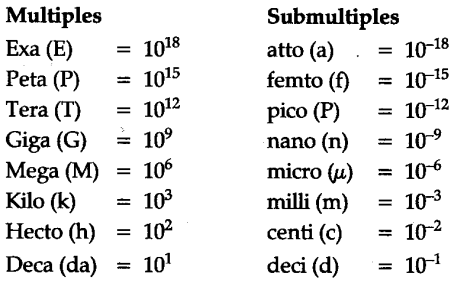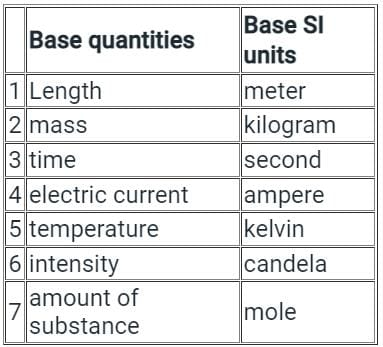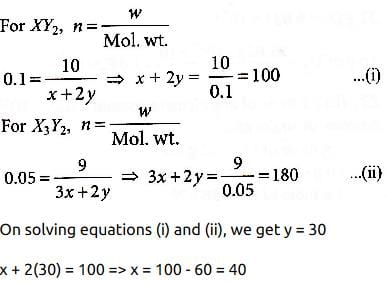NEET  >  Test: Importance & Scope Of Chemistry

# Test: Importance & Scope Of Chemistry

Test Description

## 20 Questions MCQ Test Topic-wise MCQ Tests for NEET | Test: Importance & Scope Of Chemistry

Test: Importance & Scope Of Chemistry for NEET 2022 is part of Topic-wise MCQ Tests for NEET preparation. The Test: Importance & Scope Of Chemistry questions and answers have been prepared according to the NEET exam syllabus.The Test: Importance & Scope Of Chemistry MCQs are made for NEET 2022 Exam. Find important definitions, questions, notes, meanings, examples, exercises, MCQs and online tests for Test: Importance & Scope Of Chemistry below.
Solutions of Test: Importance & Scope Of Chemistry questions in English are available as part of our Topic-wise MCQ Tests for NEET for NEET & Test: Importance & Scope Of Chemistry solutions in Hindi for Topic-wise MCQ Tests for NEET course. Download more important topics, notes, lectures and mock test series for NEET Exam by signing up for free. Attempt Test: Importance & Scope Of Chemistry | 20 questions in 20 minutes | Mock test for NEET preparation | Free important questions MCQ to study Topic-wise MCQ Tests for NEET for NEET Exam | Download free PDF with solutions
 1 Crore+ students have signed up on EduRev. Have you?
Test: Importance & Scope Of Chemistry - Question 1

### The prefix used for 10-18 is:

Detailed Solution for Test: Importance & Scope Of Chemistry - Question 1

The prefix used for 10-18 is atto-

Additional Information:metric prefix is a unit prefix that precedes a basic unit of measure to indicate a multiple or submultiple of the unit. Each prefix has a unique symbol that is prepended to any unit symbol. Some important prefixes are given below:Test: Importance & Scope Of Chemistry - Question 2

### One atomic mass unit (amu) is closest to:​

Detailed Solution for Test: Importance & Scope Of Chemistry - Question 2
• An atomic mass unit (symbolized AMU or amu) is defined as precisely 1/12 the mass of an atom of carbon-12.
• The carbon-12 (C-12) atom has six protons and six neutrons in its nucleus.
• In imprecise terms, one AMU is the average of the proton rest mass and the neutron rest mass.
• 1 AMU = 1.67377 x 10 -27 kilogram or 1.67377 x 10 -24 gram.
Test: Importance & Scope Of Chemistry - Question 3

### The number of base SI units is:

Detailed Solution for Test: Importance & Scope Of Chemistry - Question 3

The standard units of measurement defined by the ISU for the seven base quantities are SI base units All other SI units are derived from it.

Additional Information: 7 Basic SI units with their quantities are:Test: Importance & Scope Of Chemistry - Question 4

The SI unit of time is:

Detailed Solution for Test: Importance & Scope Of Chemistry - Question 4

The S.I. unit of time is second(s).
Additional Information: A second can be defined as  1/86400th part of a mean solar day,  i.e., =1/86400 × one mean solar day.

Test: Importance & Scope Of Chemistry - Question 5

The two effective drugs which act as life-saving drugs for cancer therapy and AIDS victims respectively are:

Detailed Solution for Test: Importance & Scope Of Chemistry - Question 5
• Cisplatin is particularly effective against testicular cancer.
• Taxol (paclitaxel) is a mitotic inhibitor used in cancer chemotherapy.
• AZT is used for helping AIDS victims.

Additional Information: Its mode of action has been linked to its ability to crosslink with the purine bases on the DNA; interfering with DNA repair mechanisms, causing DNA damage, and subsequently inducing apoptosis in cancer cells.

Test: Importance & Scope Of Chemistry - Question 6

Which of the following statements is/are correct?

Detailed Solution for Test: Importance & Scope Of Chemistry - Question 6
• A molecule is made up of atoms bonded together.
• The law of definite proportions, sometimes called Proust's law, or the law of constant composition states that a given chemical compound always contains its component elements in a fixed ratio (by mass) and does not depend on its source and method of preparation.

Since both statements A and B are correct, the correct option is D.

Test: Importance & Scope Of Chemistry - Question 7

The coefficient in 6.65 × 104 is:

Detailed Solution for Test: Importance & Scope Of Chemistry - Question 7

Here, 6.65 is the coefficient and 10is the exponent.

Explanation: A coefficient is a number or an alphabet that is multiplied by a variable of a single term or the terms of a polynomial. For example, in the term 7x, 7 is the coefficient.

Test: Importance & Scope Of Chemistry - Question 8

The ‘Candela’ is the unit of:

Detailed Solution for Test: Importance & Scope Of Chemistry - Question 8
• Luminous intensity is a measure of the wavelength-weighted power emitted by a light source in a particular direction per unit solid angle.
• The SI unit of luminous intensity is the candela, an SI base unit.
Test: Importance & Scope Of Chemistry - Question 9

The number of significant figures in 3256 is:

Detailed Solution for Test: Importance & Scope Of Chemistry - Question 9
• The significant figures of numbers are digits to convey the meaning that contributes to its measurement resolution.
• Non-zero digits are always significant.
• Here, 3256, this number has four non-zero numbers.
• That's why it has four significant figures.
Test: Importance & Scope Of Chemistry - Question 10

The SI unit of electric current is:

Detailed Solution for Test: Importance & Scope Of Chemistry - Question 10
• Electric current is defined as the rate of flow of charge
• Since the charge is measured in coulombs and time in seconds, the electric current unit is Coulomb/Sec ( C/s )
• Where, coulomb/sec is defined as Ampere

So the Ampere is the SI unit of the current.

Test: Importance & Scope Of Chemistry - Question 11

A number of significant figures in 0.001520 is:

Detailed Solution for Test: Importance & Scope Of Chemistry - Question 11
• The significant figures of numbers are digits to convey the meaning that contributes to its measurement resolution.
• Non-zero digits are always significant.
• Here, the number of Significant figures in 0.001520 is 4.
Test: Importance & Scope Of Chemistry - Question 12

If the true value for a result is 2.00 g and a student takes 2 measurements and reports the results as 1.95 g and 1.93 g, then we can conclude that:​

Detailed Solution for Test: Importance & Scope Of Chemistry - Question 12
• Precision indicates how closely repeated measurements match each other.
• Since both the values are close to each other, they are precise and since there is a difference between the mean value and true value, they are not accurate.
Test: Importance & Scope Of Chemistry - Question 13

The unit of amount of substance is:

Detailed Solution for Test: Importance & Scope Of Chemistry - Question 13
• The mole, denoted by the symbol mol, is the SI unit of the amount of substance.
• One mole contains exactly 6.022 140 76 x 1023 elementary entities.
• This number is the fixed numerical value of the Avogadro constant, NA, when expressed in the unit mol–1 and is known as the Avogadro number.
• The value of Avogadro’s number is fixed and the value is NA = 6.022 x 1023.
Test: Importance & Scope Of Chemistry - Question 14

Out of the numbers: 6.26, 5.8, 0.00267, 0.03, the one with the least significant figures is:​

Detailed Solution for Test: Importance & Scope Of Chemistry - Question 14
• The significant figures of numbers are digits to convey the meaning that contributes to its measurement resolution.
• Non-zero digits are always significant.
• 6.26 & 0.00267 contains 3 significant figures while 5.8 has two significant figures and 0.03 contains least that is 1.

Therefore, the correct answer is 0.03

Test: Importance & Scope Of Chemistry - Question 15

If the true value for a result is 3.00 m and a student records two readings as 3.01 m and 2.99 m, then we can conclude that:​

Detailed Solution for Test: Importance & Scope Of Chemistry - Question 15
• Precision indicates how closely repeated measurements match each other.
• Accuracy indicates how closely a measurement matches the correct or expected value.

Since the readings closely match the result and the expected value, the correct answer is that the values are both accurate and precise.

Test: Importance & Scope Of Chemistry - Question 16

The number of significant figures in 3.1205 are:

Detailed Solution for Test: Importance & Scope Of Chemistry - Question 16

The answer is 5 because all non zero digits and zero between the non zero digits are significant.

Test: Importance & Scope Of Chemistry - Question 17

The scientific notation for 0.00016 is:​

Detailed Solution for Test: Importance & Scope Of Chemistry - Question 17

Move the decimal point to the right of 0.00016 up to 4  places.

The decimal point was moved 7 places to the right to form the number 1.6

Since the numbers are less than 10 and the decimal is moved to the right, so we use a negative exponent here.

⇒ 0.00016 = 1.6 × 10-4

Explanation:

• Scientific notation is a form of presenting very large numbers or very small numbers in a simpler form.
• Scientific notation is used to represent the numbers that are very huge or very tiny in a form of multiplication of a single-digit number and 10 raised to the power of the respective exponent.
• The exponent is positive if the number is very large and it is negative if the number is very small.
Test: Importance & Scope Of Chemistry - Question 18

Suppose the elements X and Y combine to form two compounds XY2 and X3Y2. When 0.1 mole of XY2 weighs 10 g and 0.05 mole of X3Y2 weighs 9 g, the atomic weights of X and Y are:

Detailed Solution for Test: Importance & Scope Of Chemistry - Question 18

Let atomic weight of element X is x and that of element Y is y.Test: Importance & Scope Of Chemistry - Question 19

If we add the numbers 6.68 and 0.8, the result will be:​

Detailed Solution for Test: Importance & Scope Of Chemistry - Question 19

The correct option is B

7.5

In addition, the result cannot have more digits to the right of the decimal point than either of the original numbers.

Since there is only 1 digit after the decimal point in 0.8 so the result 7.48 should be rounded off to 1 decimal point i.e. 7.5.

Test: Importance & Scope Of Chemistry - Question 20

Molecular mass of glucose molecule (C6H12O6) is:

Detailed Solution for Test: Importance & Scope Of Chemistry - Question 20

Molecular mass: The sum of the atomic masses of all the atoms in a molecule of a substance is called the molecular mass of the molecule.

Generally, we use relative atomic masses of atoms for calculating the molecular mass of 1 mole of any molecular or ionic substances.

The molecular formula of glucose is C6H12O6

• The atomic mass of H = 1
• The atomic mass of C = 12
• The atomic mass of O = 16

Molecular mass of C6H12O6 = 12 (Atomic mass of Hydrogen) + 6(Atomic mass of carbon) + 6(Atomic mass of oxygen)

= 12 × 1 + 6 × 12 + 6 × 16

= 12 + 72 + 96 = 180 u.

## Topic-wise MCQ Tests for NEET

1493 tests
 Use Code STAYHOME200 and get INR 200 additional OFF Use Coupon Code
Information about Test: Importance & Scope Of Chemistry Page
In this test you can find the Exam questions for Test: Importance & Scope Of Chemistry solved & explained in the simplest way possible. Besides giving Questions and answers for Test: Importance & Scope Of Chemistry, EduRev gives you an ample number of Online tests for practice

## Topic-wise MCQ Tests for NEET

1493 tests

### How to Prepare for NEET

Read our guide to prepare for NEET which is created by Toppers & the best Teachers